Open in App
Not now

# Mensuration

• Difficulty Level : Basic
• Last Updated : 20 Jan, 2023

Mensuration is the branch of geometry that deals with the measurement of area, length, or volume in 2D and 3D shapes. The 2D shapes can be drawn in a plane like square, rectangle, triangle, circle, etc. and 3D shapes cannot be represented in a plane like bricks, ice-cream cones, football, etc. Mensuration includes computation using mathematical formulas and algebraic equations. Lets us learn about mensuration in detail in this article.

## What is Mensuration?

Mensuration is measuring various parameters of 2-D and 3-D shapes. We see various 3-D shapes in our daily life whose parameters we have to find. So, mensuration formulas are deduced to solve our problems. When learning to measure objects we learn to do so for both 3D shapes and 2D shapes. Various mensuration formulas for 2-D and 3-D shapes are calculated which help us to solve various problems. With the help of mensuration formulas, we can find the area, perimeter, and volume of various objects.

## Types of Geometrical Shapes

Geometrical shapes of various types are broadly categorised into two different categories.

• 2-Dimensional Shapes
• 3-Dimensional Shapes

## 2-Dimensional Shapes

In 2D objects have only two dimensions, such as width and height but no thickness. Like a square, rectangle, triangle, or circle. In mathematical representation, it has Two-axis (X and Y). Having only two-axis and no thickness, these 2D objects do not exist in the real world and can be represented only by using plain surfaces.

Examples of various 2-D shapes are given below in this image.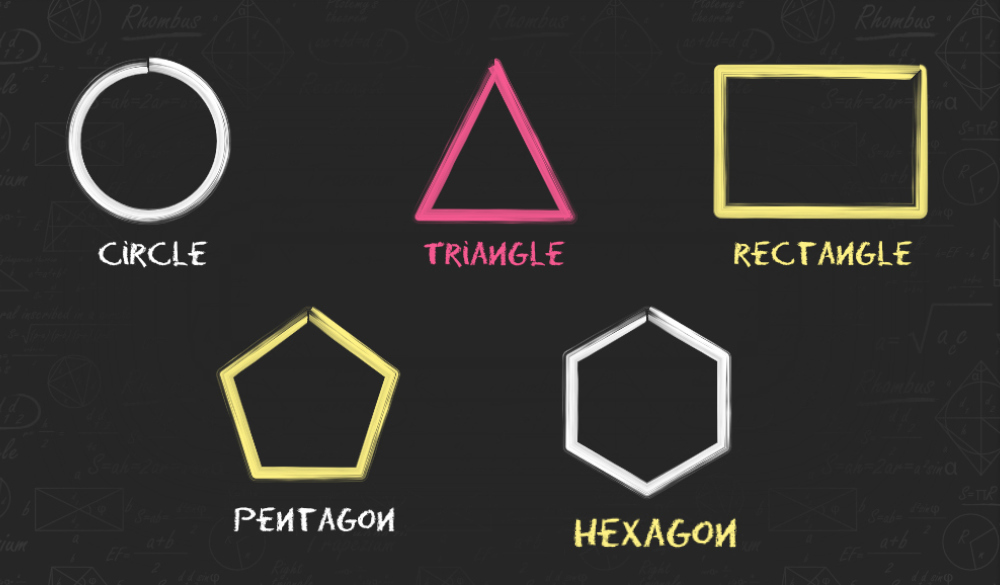## 3-Dimensional Shapes

In 3D objects have three dimensions (such as height, width, and depth), like any object in the real world. In mathematical representation, it has three-axis (X, Y, and Z). Unlike 2D shapes, 3D shapes have more parameters to cover. 3D objects have some volume and Total Surface area that uses all three dimensions i.e. length, width, and depth of the object.

Examples of various 3-D shapes are given below in this image.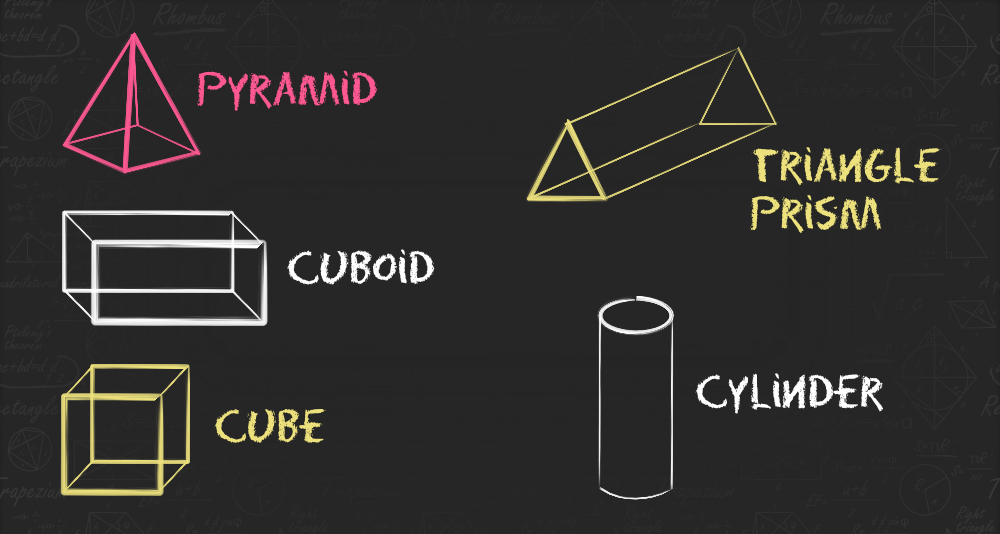## Mensuration Formulas

Mensuration formulas involve both 3D and 2D shapes. The most commonly used formula is the surface area and volume of these shapes. However, let us learn all the formulas for these shapes.

## Formulas for 2D shapes

Formulas for the surface area and volume of 2D Shapes are discussed below in this article.

## Rectangle

A rectangle is a 2D shape, having 4 sides and 4 corners. The rectangle is a quadrilateral with four right angles, so, each angle is 90°. The sum of all the interior angles is equal to 360 degrees. The opposite sides are parallel and equal to each other. Diagonals of a rectangle have the same length.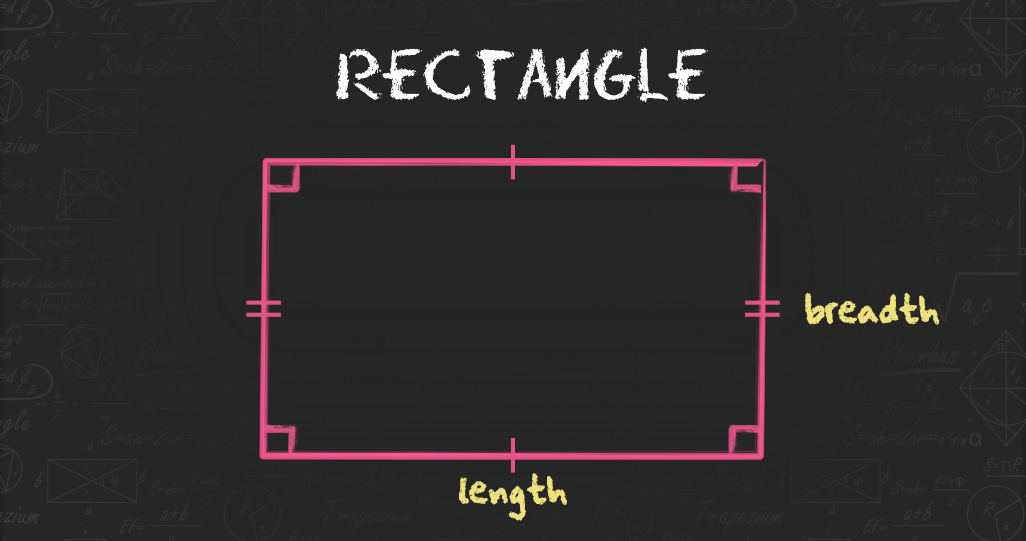Perimeter of a Rectangle = 2(Length+Breadth)

Area of a Rectangle = Length×Breadth

For more detail, check

## Square

A square is a 2D shape plane figure with four equal sides and all four angles are equal to 90 degrees. Diagonals of a square are of equal length.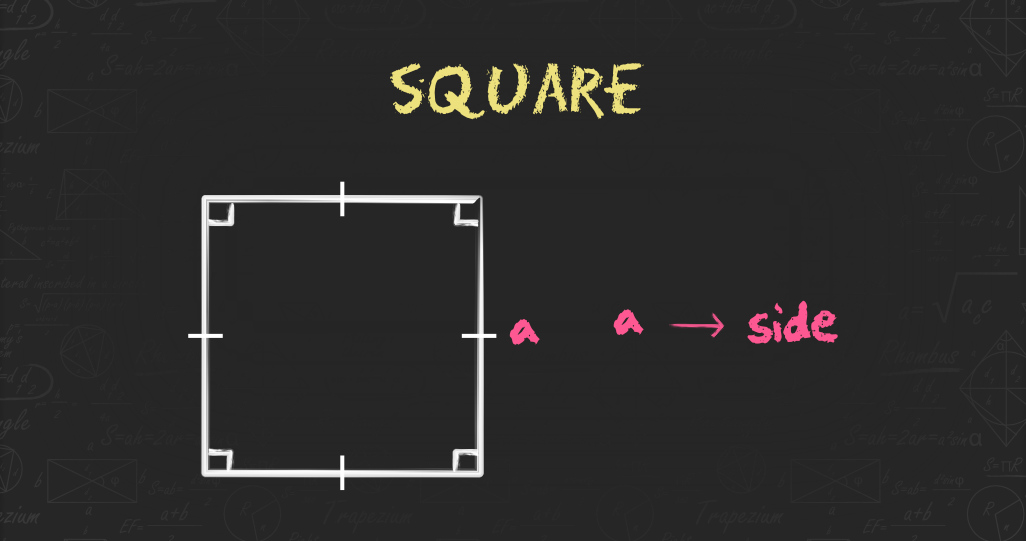Area of a Square= Side2

Perimeter of a Square= 4(Side)

For more detail, check

## Circle

A circle is a basic 2D shape, and it is a set of points in a plane that are equidistant from the centre. The distance between the centre and any point on the circumference is called the radius.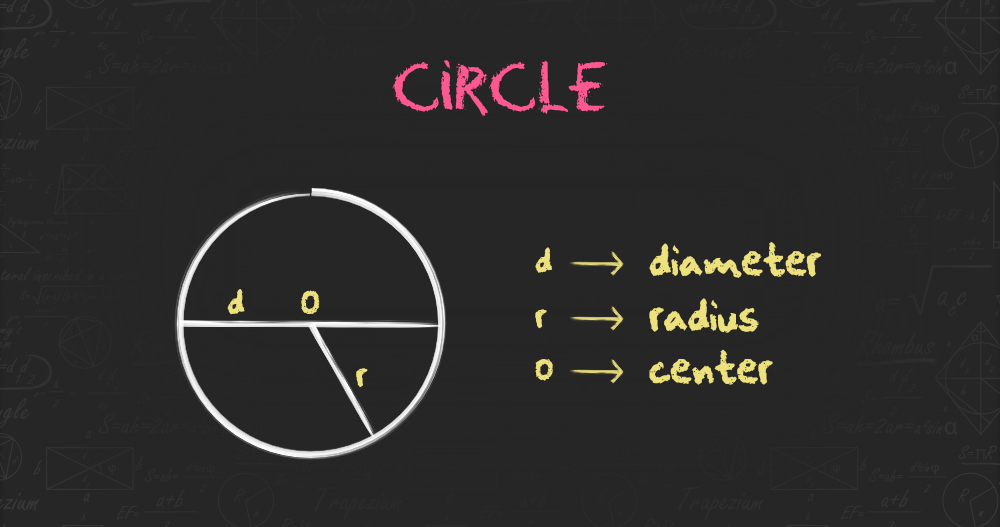Diameter of a Circle = 2 × Radius

Circumference of a Circle = π × Diameter or 2 × π × Radius

Area of a Circle = π × Radius2

For more detail, check

## Triangle

A triangle has three sides and three inclusive angles. All three angles of a triangle always add up to 180°.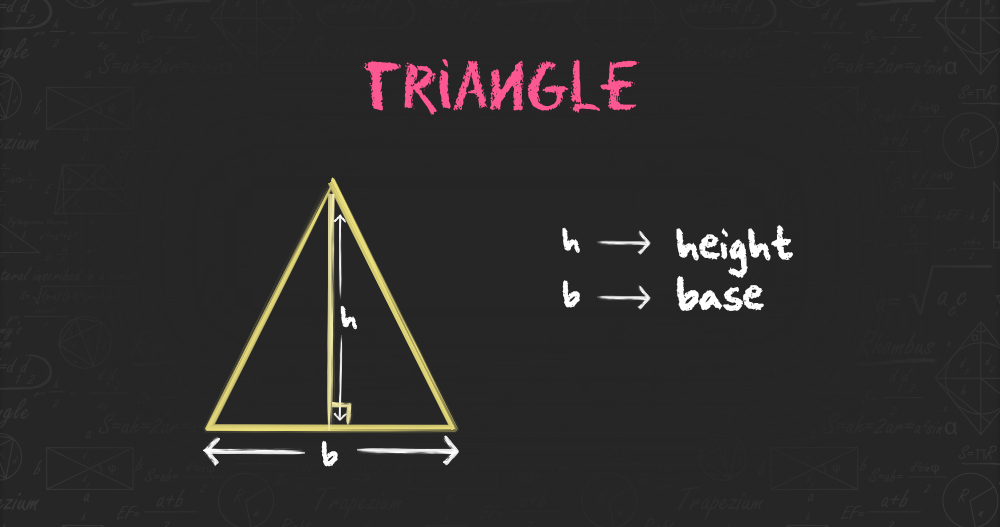Area of a Triangle = ½ × b × h

For more detail, check

## Parallelogram

A parallelogram is a 2D shape whose opposite sides are parallel to each other, It has four sides, where the pair of parallel sides are equal in length.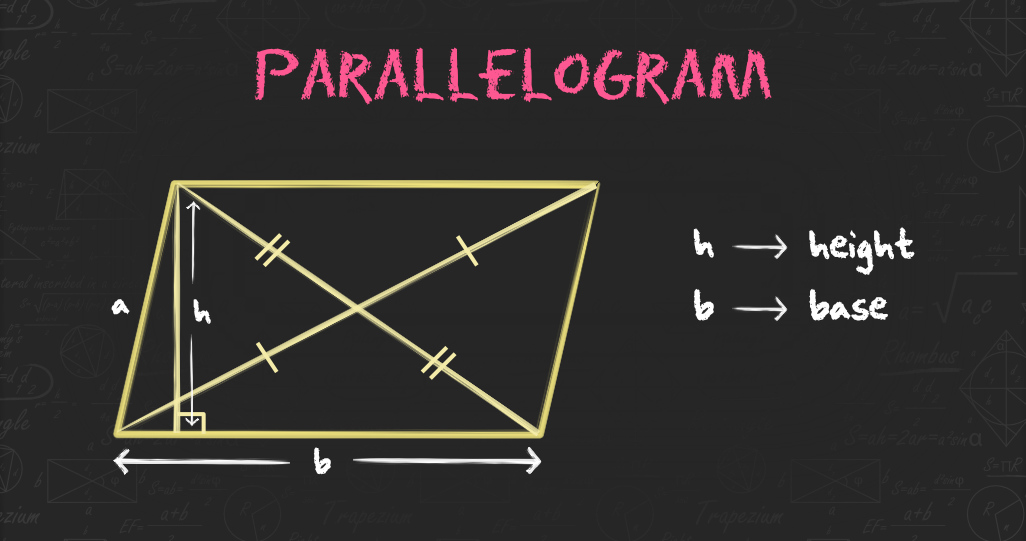Perimeter of a Parallelogram = 2 (a+b)

Area of a Parallelogram = b × h

For more detail, check

## Formulas for 3D Shapes

Formulas for the surface area and volume of 3D Shapes are discussed below in this article.

## Cube

Cube is a solid 3D figure, which has 6 square faces, 8 vertices, and 12 edges, such that 3 edges meet at one vertex point. An example of a cube is a piece of Sugar or ice with six square sides.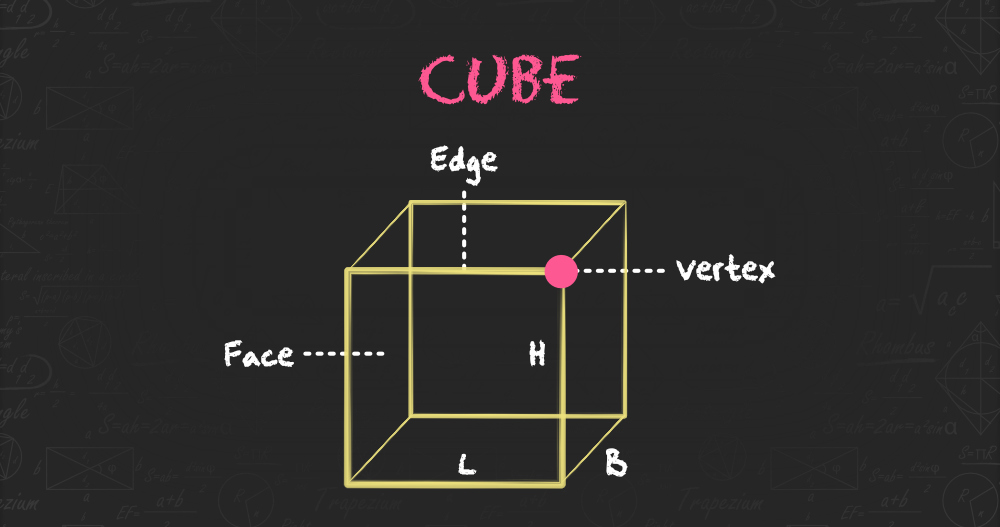Volume of a Cube = Side3 cubic units.

Lateral Surface Area of a Cube= 4 × side2 sq.units.

Total Surface Area of a Cube= 6× side2 sq. units.

For more details check,

## Cuboid

A cuboid is a 3D figure with three sides where all the sides are not equal. All of its faces are rectangles having a total of 6 faces, 8 vertices, and 12 edges.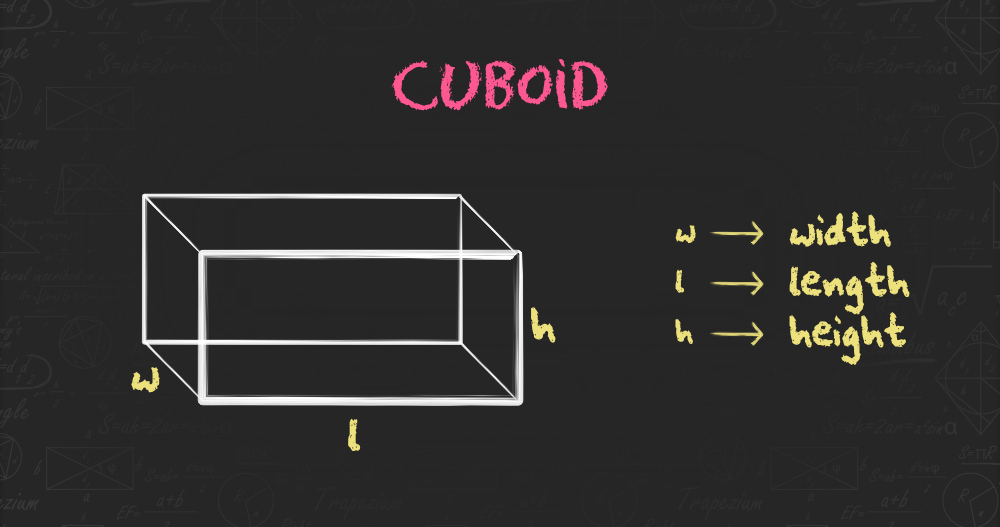Volume of a Cuboid = (length+width+height) cubic units.

Lateral Surface Area of a Cuboid = 2×height (length + width) sq. units.

Total Surface Area of a Cuboid = 2(length × width + length × height + height × width) sq.units.

Diagonal length of a Cuboid = length2 + breadth2 + height2 units.

For more details check,

## Sphere

A sphere is an object that is an absolutely round geometrical shape in 3D space. It is the set of all points in a space equidistant from a given point called the centre of the sphere. The distance between any point of the sphere and its centre is called the radius(R).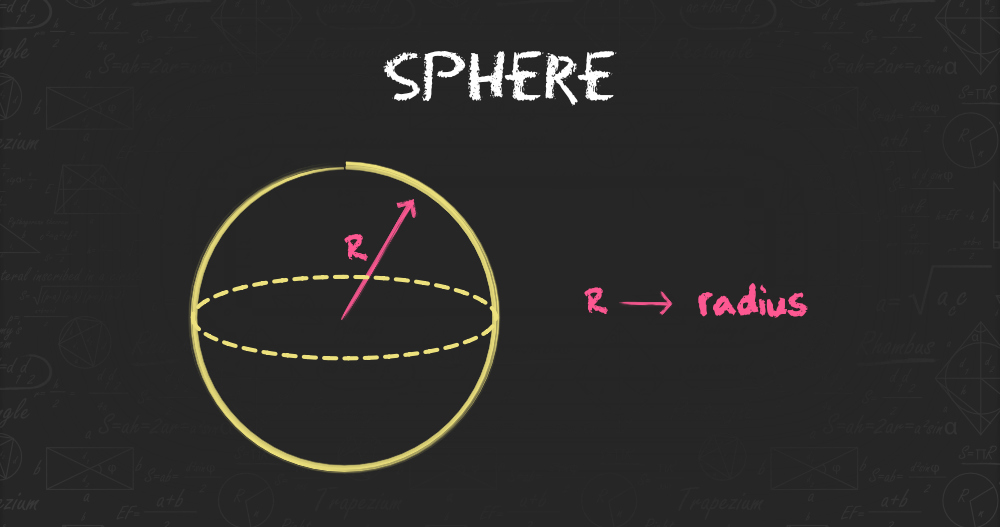Volume of a Sphere = 4/3 x π x radius³ cubic units.

Surface Area of a Sphere = 4x π x radius² sq. units.

For more details check,

## Cone

A cone is a three-dimensional geometric shape. It is formed by using a set of line segments or the lines which connect a common point, called the apex or vertex. The base of a cone it has circular, so we can compute the value of the radius. And the length of the cone from the apex to any point on the circumference of the base is the slant height.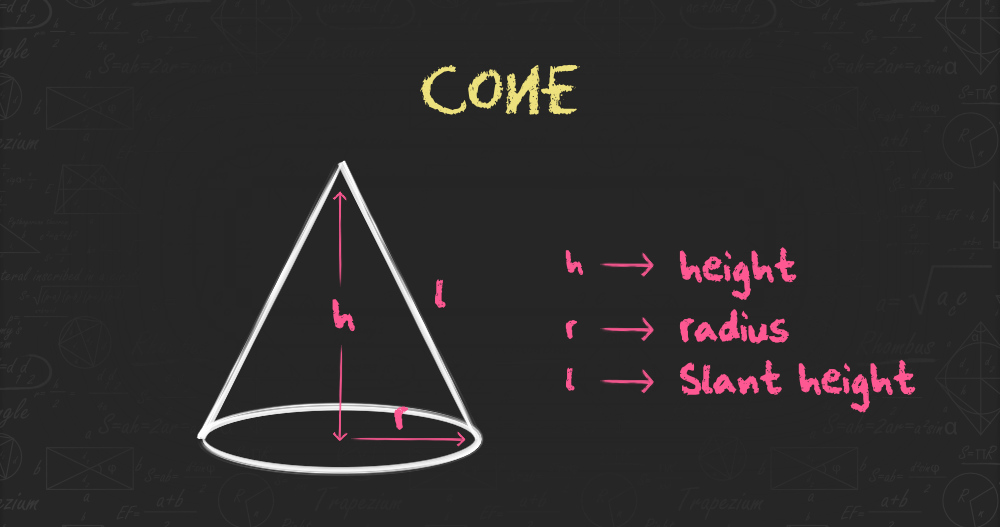Volume of a Cone = 1/3 × × π × radius² × height cubic units.

Total Surface Area of the Cone = πr(l + radius)

For more details check,

## Uses of Mensuration

Mensuration is an important topic with high applicability in real-life scenarios. Given below are some of the scenarios:

1. Measurement of agricultural fields, and floor areas required for purchase/selling transactions.
2. Measurement of volumes required for packaging milk, liquids, and solid edible food items.
3. Measurements of surface areas required for estimation of painting houses, buildings, etc.
4. Volumes and heights are useful in knowing water levels and amounts in rivers or lakes.
5. Optimum cost packaging sachets for milk etc. like tetra packing.

## Solved Examples on Mensuration

Example 1: Find the area of a square with a side of 15 cm.

Solution:

Area of a square = side × side

Here, side = 15 cm

Area = 15 × 15= 225

Therefore, the area of the square = 225 cm2

Example 2: Find the area and perimeter of a square whose side is 25 cm.

Solution:

Side = a = 25 cm

Area of a square = a2 square units

Area of a square = 252

Area of square = 25 x 25
= 625

Therefore, area of a square = 625 cm2

Perimeter of a square = 4a units

P = 4 x 25 =100

Therefore, perimeter of a square = 100 cm

Example 3: Find the surface area of a cuboid with a length of 3 units, a width of 4 units, and a height of 5 units.

Solution:

Length of the cuboid = 3 units
Breadth of the cuboid = 4 units
Height of the cuboid = 5 units

Surface Area of cuboid = 2 × (lb + bh + lh) square units

= 2[(3 × 4) + (4 × 5) + (3 × 5)]

= 2(12 + 20 + 15)

= 2(47)

= 94 square units

Therefore, surface area of the cuboid is 94 square units.

Example 4: Find the area and circumference of a circle whose radius is 7 cm.

Solution:

Radius of circle = 7 cm

Area of a circle = π × r2   (π = 22/7)

= 22/7 × 7 × 7

= 154 cm2

Therefore, Area of the circle = 154 square cm

Circumference of a circle = 2πr

= 2 × (22/7) × 7

= 44 cm

Therefore, circumference of the circle is 44 cm

## FAQs on Mensuration

### Question 1: Who Introduced Mensuration?

Archimedes was credited for the introduction of mensuration. He is one of the greatest mathematicians of the ancient era. He was the first to formulate the perimeter and the area of various 2-D shapes.

### Question 2: What is mensuration in Maths?

Mensuration in mathematics is defined as the study of the measurement such as the area and perimeter of various 2D and 3D geometric shapes.